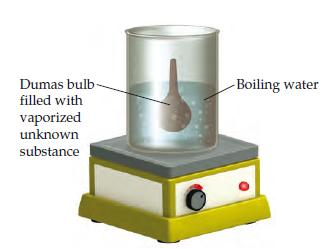# Problem: The molar mass of a volatile substance was determined by the Dumas-bulb method. (In the Dumas-bulb technique for determining the molar mass of an unknown liquid, you vaporize the sample of a liquid that boils below 100oC in a boiling-water bath and determine the mass of vapor required to fill the bulb (see drawing ). The unknown vapor had a mass of 0.847 g, the volume of the bulb was 355 cm3, pressure was 753 torr, and temperature was 100 oC.Calculate the molar mass of the unknown vapor.

###### FREE Expert Solution

We are asked to calculate the molar mass of the unknown vapor.

Given:

m = 0.847 g

V = 355 cm= 0.355 L

P = 753 torr = 0.991 atm

T = 100 oC +273.15 = 373.15 K

R = 0.08206 L-atm/mol-K

80% (147 ratings)###### Problem Details

The molar mass of a volatile substance was determined by the Dumas-bulb method. (In the Dumas-bulb technique for determining the molar mass of an unknown liquid, you vaporize the sample of a liquid that boils below 100oC in a boiling-water bath and determine the mass of vapor required to fill the bulb (see drawing). The unknown vapor had a mass of 0.847 g, the volume of the bulb was 355 cm3, pressure was 753 torr, and temperature was 100 oC.

Calculate the molar mass of the unknown vapor.

Frequently Asked Questions

What scientific concept do you need to know in order to solve this problem?

Our tutors have indicated that to solve this problem you will need to apply the The Ideal Gas Law: Molar Mass concept. You can view video lessons to learn The Ideal Gas Law: Molar Mass. Or if you need more The Ideal Gas Law: Molar Mass practice, you can also practice The Ideal Gas Law: Molar Mass practice problems.